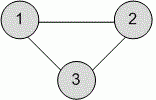Competitions

# Complete graph

Undirected graph is called complete, if any pair of its different vertices is connected with at least one edge. For a given list of graph edges, check whether it is complete.

#### Input

The first line contains the number of vertices n (1n100) and the number of edges m (1m10000) in the graph. Then m pairs of numbers are given - the graph edges.

#### Output

Print YES if the graph is complete and NO otherwise.Time limit 1 second
Memory limit 128 MiB
Input example #1
3 3
1 2
1 3
2 3

Output example #1
YES

Input example #2
5 18
1 2
1 3
1 3
1 4
1 4
1 4
1 5
1 5
2 3
2 4
2 4
2 5
3 4
3 4
3 4
3 5
3 5
4 5

Output example #2
YES